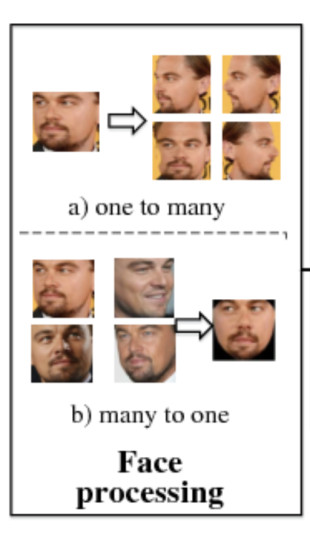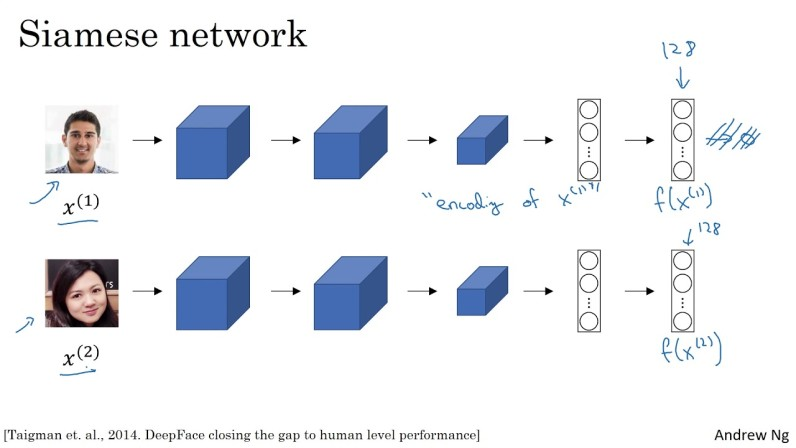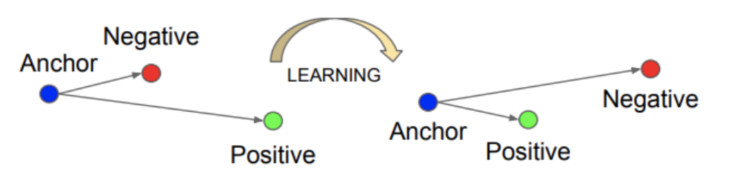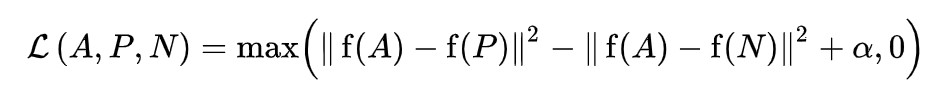Sept. 24, 2019

# An overview of Face Recognition

So far we have seen face detection to localize faces in images and align these faces to remove the background and improve the recognition. Now we will see how we can use a face recognition system to identify the people presented in these images.

• Face detection: Localize faces in images or videos.

• Face recognition: We can categorize this task as:

• Face verification: Determines if the person in two images is the same. (one to one)

• Face identification: Determines if the person in one image appears in one of the images of the database. (one to many)

## Face recognition components

Before using the aligned faces to identify the people, we can perform some steps to get a higher accuracy and increase the security:

#### Face Anti-spoofing

Thanks to Face Anti-spoofing we can recognize if the face is live or spoofed in order to avoid different types of attacks.

#### Face Processing

To increase the performance of the face recognition system we can use two face processing methods:

• One-To-Many Augmentation: We generate multiple images with different poses from a single image. Due to this, the network can learn pose-invariant representations.

• Many-To-One Normalization: Here we recover the canonical views (front, top and side views of an object, in this case the face) from one or multiple images of a non-frontal view.In the face recognition world there are multiple architectures that have improved through the years. The best architectures that we can find are based on convolutional neural networks. In order to understand these architectures we have to learn an important concept called N-shot learning.

## N-shot learning

N-shot learning is a training technique where we only have N examples of each class. Due to this, we can train a neural network with few examples and multiple classes. One popular architecture that uses this technique are the Siamese Neural Networks which can be trained by only using one example for each class.

Since we only use a few examples of each class to compute the training process, the neural networks do not classify the image directly, instead they use the embedding of the last layer before the classification layers, this embedding is a vector that contains the features of the input image in the euclidean space

In other words, the neural network maps the input image into the metric space, in this space if two images belong to the same class the distance between them is short whereas if the images are from different classes the distance is greater.

## FaceNet

FaceNet is a convolutional neural network architecture used in face recognition introduced by Google. FaceNet is based on siamese networks, the siamese network is used two or more times to get embeddings from different faces.

FaceNet used the GoogleNet-24 architecture to build the siamese network and was trained in a private dataset.Along with this architecture, triplet loss was also introduced. In this loss function we have three embeddings from face images, an anchor, a positive and a negative, the anchor and the positive are two embeddings of the same person and the negative of a different person. Thus, the loss function minimizes the Euclidean distance between the anchor and the positive and maximizes the Euclidean distance between the anchor and the negative.We can define the Euclidean distance as the straight-line distance between two points in the Euclidean space. Triplet loss uses the l2 norm (also called l2 distance), a norm or magnitude is just the length or size of a vector. The euclidean norm is the l2 norm.Triplet loss function (Image from https://en.wikipedia.org/wiki/Triplet_loss)

``````
||f(A) - f(P)||2

``````

Means the l2 distance or norm between the anchor embedding and positive embedding. The l2 distance is calculated as the square root of the sum of the squared vector values.

We use the max function to indicate the loss, if the distance between the anchor and positive:

``````
||f(A) - f(P)||2

``````

is greater than the distance between the anchor and negative:

``````
||f(A) - f(N)||2

``````

for example:

``````
||f(A) - f(P)||2 = 10

||f(A) - f(N)||2 = 5

max(10 - 5, 0)

max(5, 0)

``````

we have a loss of 5, whereas if the distance between the anchor and positive is less:

``````
||f(A) - f(P)||2 = 5

||f(A) - f(N)||2 = 10

max(5 - 10, 0)

max(-5, 0)

``````

We don't have loss.

In TensorFlow and Keras we can code this loss function:

``````
def triplet_loss(anchor, positive, negative, alpha):

positive_distance = tf.reduce_sum(tf.square(tf.subtract(anchor, positive)), 1)

negative_distance = tf.reduce_sum(tf.square(tf.subtract(anchor, negative)), 1)

loss = tf.reduce_mean(tf.maximum(basic_loss, 0.0), 0)

return loss

``````

We use the alpha parameter to avoid the neural network to output the same embedding that satisfy the loss function, it would be easy for the network to output always the same positive embedding which is close to the anchor and the same negative embedding which is far to the anchor. This is a simple but effective trick, if the parameter alpha has a value of 5 the loss will be 5 and the network will have to change the weights to reduce this value and at the same time this fixes the problem.

To train this neural network we need multiple images of the same person to have the anchor and the positive. The hardest part is to choose these embeddings to train the model, we need the negative to be close enough to the anchor and positive. If we choose the embeddings randomly, it would be easy to satisfy the constrain of the loss function since the distance between the negative and anchor/positive will be large most of the time.

The training process is the following one:

1. Pass the anchor image to the network and get its embedding.

2. Pass the positive image to the network and get its embedding.

3. Pass the negative image to the network and get its embedding.

4. Pass the three embeddings to the loss function.

5. Update the network parameters with respect to the loss.

The FaceNet network outputs an embedding of size 64. Once the network is trained, we can pass it face images, get its embeddings and use them to train a classifier like a support vector machine or a k nearest neighbor, the output of this classifier will be the probability of a face belonging to a certain person.

## VGGface

FaceNet is one of the most popular architectures based on convolutional neural networks used in face recognition. FaceNet was introduced back in 2015, in the same year VGGface was introduced as well, this network used the VGGNet architecture and a new dataset also called VGGface, VGGface uses the triplet loss function. The main focus of this last network was in building a large training dataset to get a better performance.

In 2017 a larger dataset called VGGface2 was introduced which presented improvements in variations like age, pose or illumination. Several models based on ResNet-50 and a SqueezeNet-ResNet-50 were trained in this dataset.

## Datasets

There are several datasets to train face recognition systems, we have to take into account some features of these datasets if we want our system to perform well.